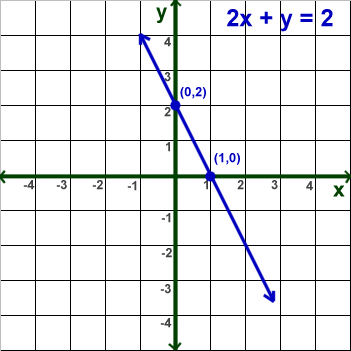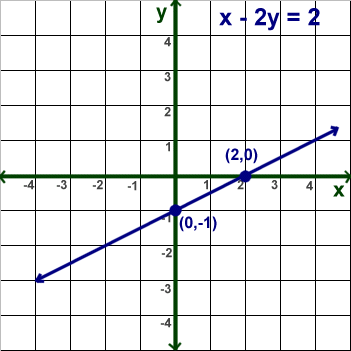Parents and Teachers: Support Ducksters by following us onor.

# Kids Math

## Intro to Linear Equations

A linear equation is an equation that describes a straight line on a graph. You can remember this by the "line" part of the name linear equation.

Standard Form

Linear equations have a standard form that looks like this:

Ax + By = C

Where A, B, and C are coefficients (numbers) while x and y are variables.

You can think of the x and y variables as points on a graph.

Example linear equations:

You can plug numbers into A, B, and C of the above standard form to make linear equations:

2x + 3y = 7
x + 7y = 12
3x - y = 1

Linear Equations Represent Lines

At first it may seem strange that an equation represents a line on a graph. To make a line you need two points. Then you can draw a line through those two points.

The x and y variables in the linear equation represent the x and y coordinates on a graph. If you plug in a number for x, you can calculate the corresponding number for y. Those two numbers show a point on a graph. If you keep plugging in numbers for x and y in a linear equation, you will find that all the points together make a straight line.

Graphing a Linear Equation

In order to graph a linear equation you can put in numbers for x and y into the equation and plot the points on a graph. One way to do this is to use the "intercept" points. The intercept points are when x = 0 or y = 0. Here are some steps to follow:
• Plug x = 0 into the equation and solve for y
• Plot the point (0,y) on the y-axis
• Plug y = 0 into the equation and solve for x
• Plot the point (x,0) on the x-axis
• Draw a straight line between the two points
You can check your answers by trying other numbers in the equation. Try x = 1. Solve for y. Then make sure that that point is on your line.

Example Problem:

Graph the linear equation: 2x + y = 2

Step 1: Plug in x = 0 and solve for y.

2 (0) + y = 2
y = 2

Step 2: Plug in y = 0 and solve for x.

2x + 0 = 2
2x = 2
x = 1

Step 3: Graph the x and y intercept points (0, 2) and (1,0)

Step 4: Draw a straight line through the two pointsWe will put in 2 for x and solve:

2(2) + y = 2
4 + y = 2
y = 2 - 4
y=-2

Is the point (2,-2) on the line?

You can try some other points to double check as well.

Example 2:

Graph the linear equation x - 2y = 2

Step 1: x = 0

0 - 2y = 2
y = -1

Step 2: y = 0

x - 2(0) = 2
x = 2

Step 3: Graph the x and y points (0, -1) and (2,0)

Step 4: Draw a line through the two pointsLet's try x = 4

4 - 2y = 2
-2y = 2 - 4
-2y = -2
2y = 2
y = 1

Is the point (4,1) on the graph?

More Algebra Subjects
Algebra glossary
Exponents
Linear Equations - Introduction
Linear Equations - Slope Forms
Order of Operations
Ratios
Ratios, Fractions, and Percentages
Solving Algebra Equations with Addition and Subtraction
Solving Algebra Equations with Multiplication and Division

Back to Kids Math

Back to Kids StudyFollow us onor